login  home  contents  what's new  discussion  bug reports help  links  subscribe  changes  refresh  edit

Recenty in sci.math.symbolic there was a question if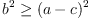(1)
and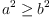(2)
implies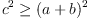(3)
or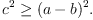(4)

This can be proved by an elementary argument. Below w show purely mechanical way based on cylindrical algebraic decomposition (CAD).

fricas
(1) -> rC := RealClosure(Fraction(Integer))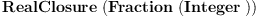(5)
Type: Type
fricas
pR := POLY(rC)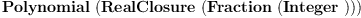(6)
Type: Type
fricas
-- Replace inequalies by differences of sides
lp := [b^2 - (a - c)^2, a^2 - b^2, c^2 - (a + b)^2, c^2 - (a - b)^2]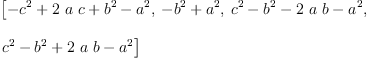(7)
Type: List(Polynomial(Integer))
fricas
-- Do CAD
cd := cylindricalDecomposition(lp::List(pR)
)\$CylindricalAlgebraicDecompositionPackage(rC)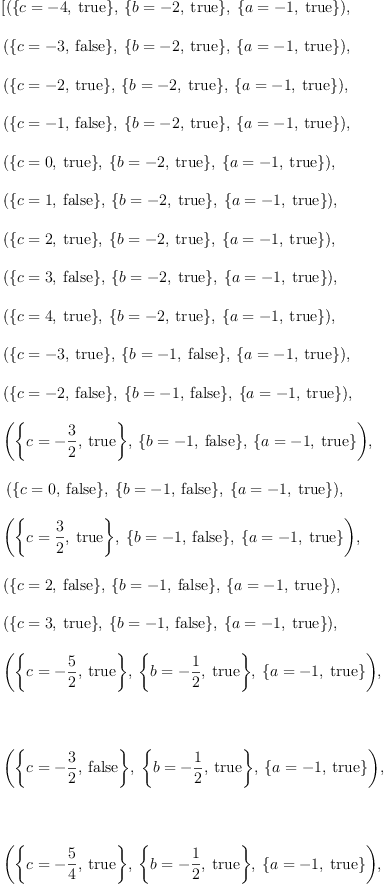(8)
Type: List(Cell(RealClosure(Fraction(Integer))))
fricas
-- If implication is false, then there is opposite strict ineqality
-- in the last two inequalities.  Hence, there is counterexample where
-- all inequalities are strict.  Consequently, it is enough to look
-- only at cells of full dimension.
cd3 := [ce for ce in cd | dimension(ce) = 3];
Type: List(Cell(RealClosure(Fraction(Integer))))
fricas
sp3 := [samplePoint(ce) for ce in cd3]::List(List(Fraction(Integer)))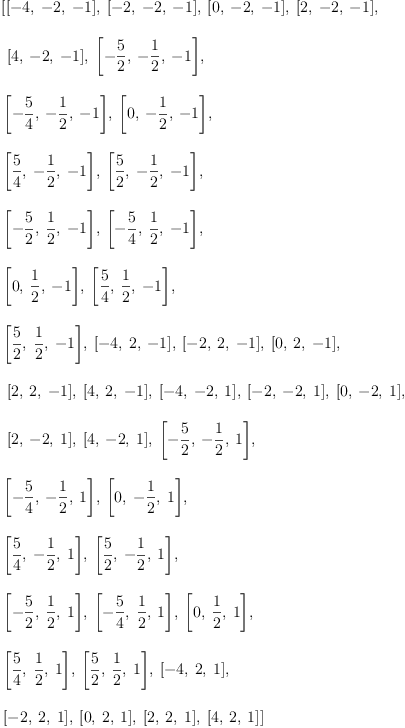(9)
Type: List(List(Fraction(Integer)))
fricas
vll := [map(p +-> eval(p, [a, b, c], pp), lp) for pp in sp3];
Type: List(List(Polynomial(Fraction(Integer))))
fricas
vlq := vll::List(List(Fraction(Integer)))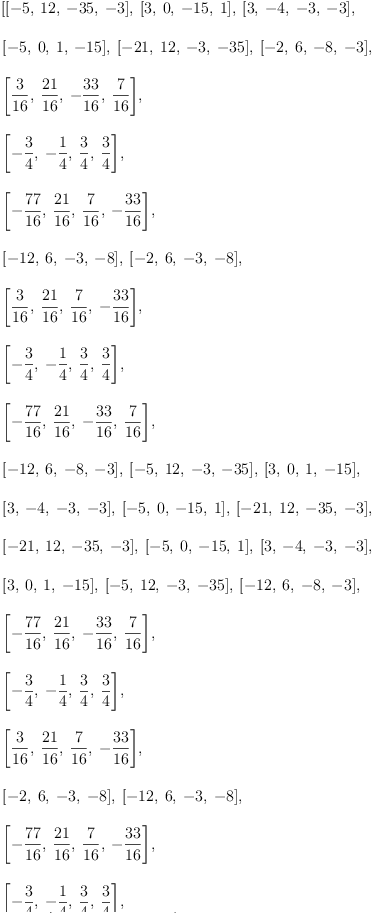(10)
Type: List(List(Fraction(Integer)))
fricas
good := true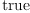(11)
Type: Boolean
fricas
-- Loop to check validity of formula at each sample point
for vl in vlq repeat
if vl(1) > 0 and vl(2) > 0 and vl(3) < 0 and vl(4) < 0 then
good := false
Type: Void
fricas
print good
true
Type: Void

Let us explain this a bit more. CAD for list of polynomials l produces collection of cells such that any boolean formula in equations and inequalites of polynomials in l produces constant value on each cells. And cells cover the whole space. So, to check validity of Boolean formula it is enough to check its validity on each cell. Since value of the formula is constant in the cell it is enough to compute value at single point given by samplePoint. Above we took advantage of another property: closure of sum of cells of full dimension gives the whole space. Since sets on which assumptions and conclusion of our formula hold are closed we can gain a little extra efficiency and ignore cells of lower dimension.

 Subject:   Be Bold !! ( 14 subscribers )